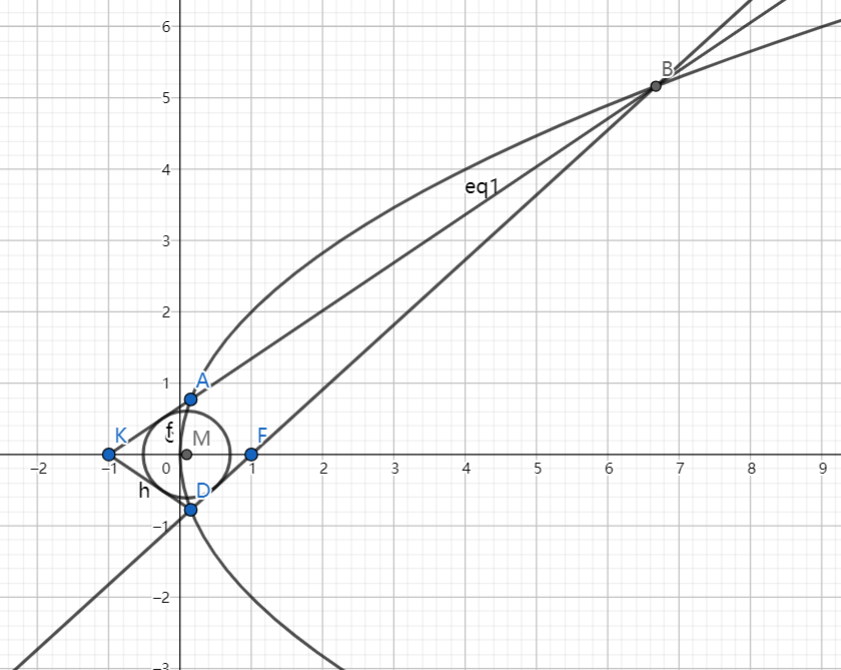# SAT1000 - P839

Geometry Level pendingAs shown above, point $F$ is the focus of the parabola $C: y^2=4x$, and line $l$ passing through $K(-1,0)$ intersects with $C$ at point $A,B$, and $A,D$ are symmetry about the x-axis.

If $\overrightarrow{FA} \cdot \overrightarrow{FB}=\dfrac{8}{9}$, then find the equation of the incircle of $\triangle BDK$.

The equation can be expressed as: $(x-a)^2+(y-b)^2=r^2$. Submit $\lfloor 1000(a+b+r) \rfloor$.

Have a look at my problem set: SAT 1000 problems

×